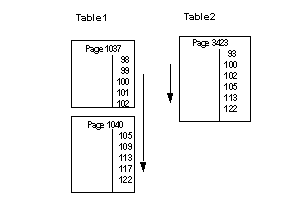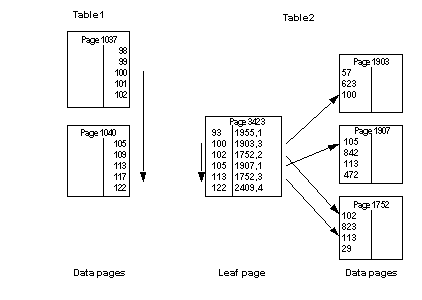# How a full-merge is performed

If both Table1 and Table2 have indexes on the join key, this query can use a full-merge join:

```select *
from Table1, Table2
where Table1.c1 = Table2.c2
and Table1.c1 between 100 and 120
```

If both tables are allpages-locked tables with clustered indexes, and Table1 is chosen as the outer table, the index is used to position the search on the data page at the row where the value equals 100. The index on Table2 is also used to position the scan at the first row in Table2 where the join column equals 100. From this point, rows from both tables are returned as the scan moves forward on the data pages.

Figure 23-3: A serial merge scan on two tables with clustered indexesMerge joins can also be performed using nonclustered indexes. The index is used to position the scan on the first matching value on the leaf page of the index. For each matching row, the index pointers are used to access the data pages. Figure 23-4 shows a full-merge scan using a nonclustered index on the inner table.

Figure 23-4: Full merge scan using a nonclustered index on the inner table